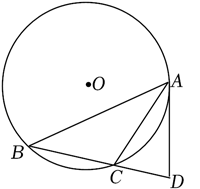(1) 求证: $A D$ 是 $\odot O$ 的切线;
(2) 若 $A C$ 是 $\angle B A D$ 的平分线, $\sin B=\frac{3}{5}, B C=4$, 求 $\odot O$ 的半径.①点击 首页查看更多试卷和试题 , 点击查看 本题所在试卷